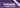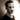More Functional Patterns: Higher-order functions and guardsContinuing our foray into the More Functional Patterns chapter, today we're looking at higher-order functions and guards.

## Higher-order functions

First off, let's establish some common ground and agree on the definition. According to Wikipedia and the Haskell wiki, a higher-order function is a function that takes other functions as arguments or returns a function as a result. In essence, it's a function that operates on functions as values. Because functions are just any other value in haskell, this comes very naturally to the language.

So to qualify as a higher-order function, a function must fulfill one of two criteria:

• Accept a function as a parameter ::

`map` and sorting functions are great examples of this. For instance, `map`'s signature is `map :: (a -> b) -> [a] -> [b]`, where the first parameter is the function used to transform the values of type `a` in the list to values of type `b`. Sorting functions could take a comparison function (`a -> a -> Ordering`) and a list (`[a]`), sort the list for you and return the sorted list.

• Return a function ::

This probably happens more than you realize. Any function that accepts more than one argument in Haskell is by default a higher-order function due to how the lambda calculus works. That said, you can also explicitly return a partially applied function based on the input arguments. For instance, this example takes a boolean value saying whether the function should return a partially applied multiplication or addition, and the first argument to apply to the calculation. It returns the partially applied function:

``````f :: Num a => Bool -> a -> (a -> a)
f multiply n =
if multiply then
(n*)
else
(n+)``````

It's a silly example, but it gets the point across.

## Guards

Next up, the book returns to a form of pattern matching by introducing guards, which allow us to run code conditionally based on the truth of a statement. If you think that sounds a lot like an `if-then-else` expression, that's because it works pretty much the same but with some different syntax. We'll run through a couple of different examples to examine the various features that are introduced.

The basic syntax looks like this:

``````myAbs :: Integer -> Integer
myAbs x
| x < 0     = (-x)
| otherwise =   x``````

This function has two guards, each beginning with a pipe symbol (`|`). Note that we don't need an equals sign after the arguments in the first line of the definition; instead, the symbol comes after each respective guard. The first of the guards that evaluates to `True` will be executed.

In this function, we have two cases: One for when the value of `x` is negative (in which case we'll return the absolute value of `x`), and one for every other case. In the above example, we have used the word `otherwise`, which is a synonym for `True`, as a catch-all for every other case.

If we want to explicitly enumerate all options, we can do that too:

``````myAbs :: Integer -> Integer
myAbs x
| x <  0 = (-x)
| x >= 0 =   x``````

This will work the exact same as the previous version, but in this case we're handling all cases explicitly. When explicitly listing all options, make sure you have enabled warnings (or errors) for non-exhaustive patterns to avoid accidentally partial functions.

What if we want to share some values between the different guards? We can use `where`-statements for that! Imagine a function that takes the lengths of the two legs (catheti) of a right triangle and returns whether the triangle is big, small, or medium based on the length of the hypotenuse:

``````triangleSize :: (Floating a, Ord a) => a -> a -> String
triangleSize c1 c2
| h >= 5 = "Big triangle"
| h >= 2 = "Medium triangle"
| h <  2 = "Small triangle"
where h = sqrt (c1^2 + c2^2)``````

When declaring variables like this, they're in scope for all of the guards.

This last example also demonstrates the importance of order on the guards. Because only the first guard that evaluates to `True` is executed, the above function works as expected. If we switched the two guards for big and medium triangles, no triangle would ever be considered 'big'. What a sad world that would be.

## Next up

That was all we had time for today, my dear reader. We still have a little bit left of this chapter: function composition and pointfree style. Two very interesting topics which I'm looking forward to covering next time. Until then: take care!

## Footnotes

 You may have heard the term 'first-class functions'. This is what that means. You can pass functions around like any other variable, store them in data structures, assign them to variables, and so forth. See MDN or Wikipedia for more.Thomas Heartman is a developer, writer, speaker, and one of those odd people who enjoy lifting heavy things and putting them back down again. Preferably with others. Doing his best to gain and share as much knowledge as possible.